Next: Application to Binomial Probability Up: Probability Theory Previous: Binomial Probability Distribution

# Mean, Variance, and Standard Deviation

What is meant by the mean, or average, of a quantity? Suppose that we wanted to calculate the average age of undergraduates at the University of Texas at Austin. We could go to the central administration building, and find out how many eighteen year-olds, nineteen year-olds, et cetera, were currently enrolled. We would then write something like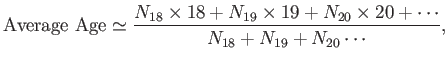(2.24)

where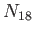is the number of enrolled eighteen year-olds, et cetera. Suppose that we were to pick a student at random and then ask What is the probability of this student being eighteen?'' From what we have already discussed, this probability is defined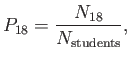(2.25)

where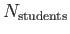is the total number of enrolled students. We can now see that the average age takes the form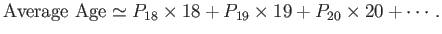(2.26)

There is nothing special about the age distribution of students at UT Austin. So, for a general variable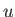, which can take on any one ofpossible values,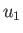,, with corresponding probabilities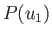,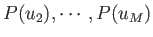, the mean, or average, value of, which is denoted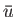, is defined as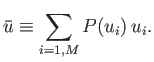(2.27)

Suppose that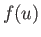is some function of. For each of thepossible values of, there is a corresponding value ofthat occurs with the same probability. Thus,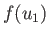corresponds to, and occurs with the probability, and so on. It follows from our previous definition that the mean value ofis given by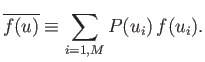(2.28)

Suppose thatand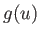are two general functions of. It follows that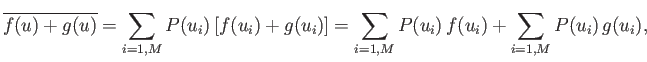(2.29)

so that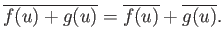(2.30)

Finally, if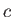is a general constant then it is clear that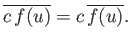(2.31)

We now know how to define the mean value of the general variable. But, how can we characterize the scatter around the mean value? We could investigate the deviation offrom its mean value,, which is denoted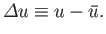(2.32)

In fact, this is not a particularly interesting quantity, because its average is obviously zero: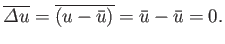(2.33)

This is another way of saying that the average deviation from the mean vanishes. A more interesting quantity is the square of the deviation. The average value of this quantity,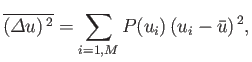(2.34)

is usually called the variance. The variance is clearly a positive number, unless there is no scatter at all in the distribution, so that all possible values ofcorrespond to the mean value,, in which case it is zero. The following general relation is often useful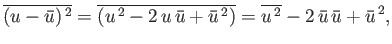(2.35)

giving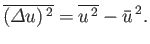(2.36)

The variance ofis proportional to the square of the scatter ofaround its mean value. A more useful measure of the scatter is given by the square root of the variance,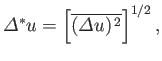(2.37)

which is usually called the standard deviation of. The standard deviation is essentially the width of the range over whichis distributed around its mean value,.Next: Application to Binomial Probability Up: Probability Theory Previous: Binomial Probability Distribution
Richard Fitzpatrick 2016-01-25